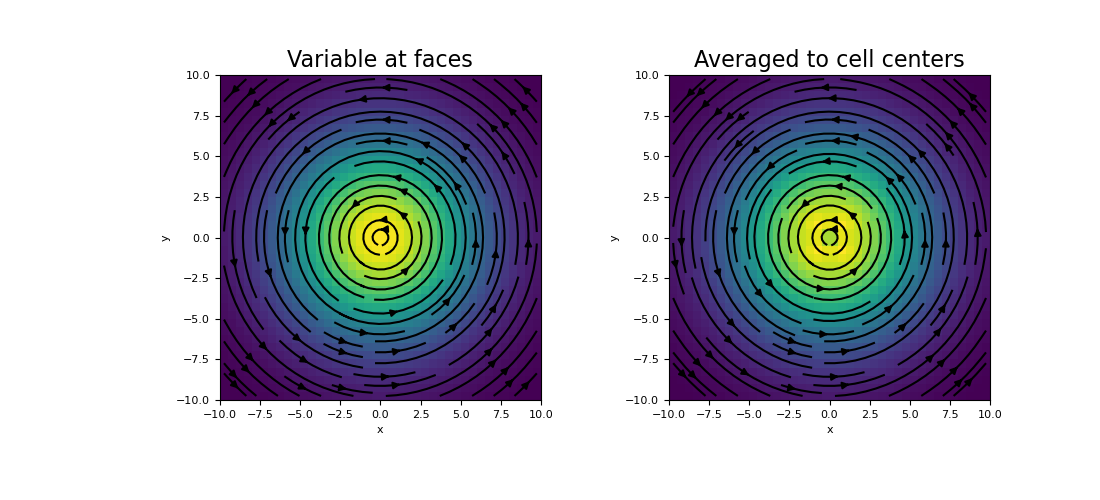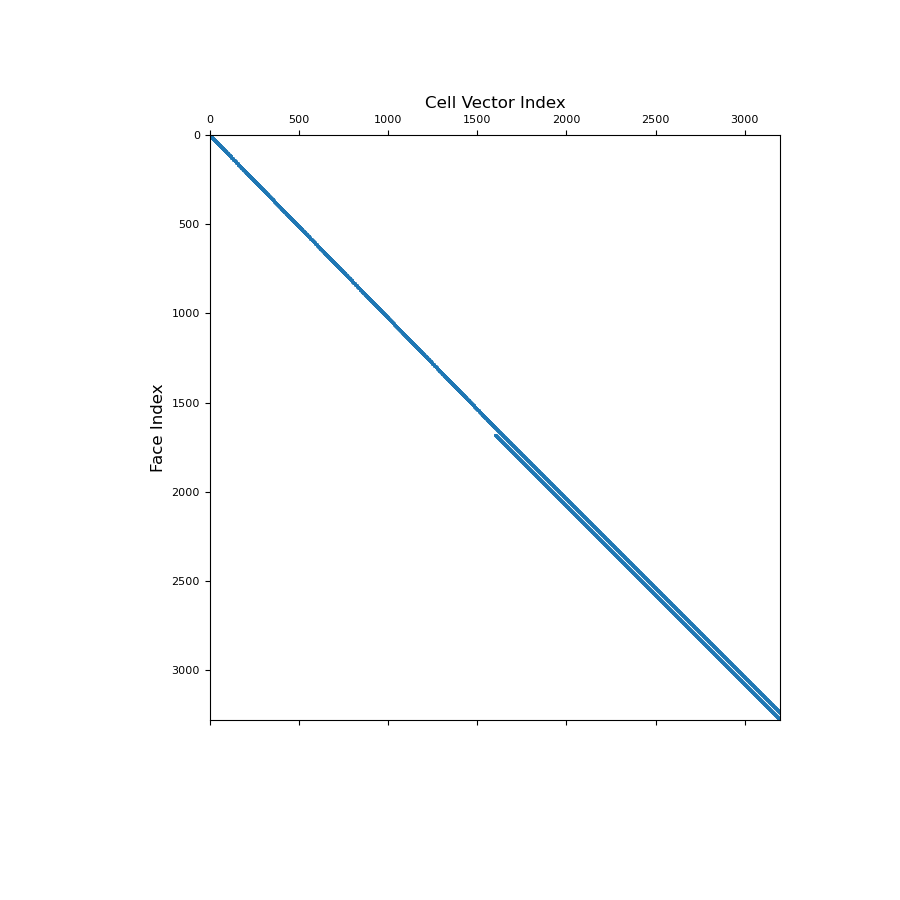# discretize.CurvilinearMesh.average_cell_vector_to_face¶

property CurvilinearMesh.average_cell_vector_to_face

Averaging operator from cell centers to faces (vector quantities).

This property constructs the averaging operator that independently maps the Cartesian components of vector quantities from cell centers to faces. This averaging operators is used when a discrete vector quantity defined at cell centers must be approximated on the faces. Once constructed, the operator is stored permanently as a property of the mesh.

Be aware that the Cartesian components of the original vector are defined seperately at cell centers in a 1D numpy.array organized [ux, uy, uz]. Once projected to faces, the Cartesian components are defined on their respective faces; e.g. the x-component lives on x-faces. The operation is implemented as a matrix vector product, i.e.:

u_f = Acf @ u_c

Returns
(n_faces, dim * n_cells) scipy.sparse.csr_matrix

The vector averaging operator from cell centers to faces. Since we are averaging a vector quantity from cell centers, the second dimension of the operator is the mesh dimension times the number of cells.

Notes

Let $$\mathbf{u_c}$$ be the discrete representation of a vector quantity whose Cartesian components are defined separately at cell centers. average_cell_vector_to_face constructs a discrete linear operator $$\mathbf{A_{cf}}$$ that projects each Cartesian component of $$\mathbf{u_c}$$ to the faces, i.e.:

$\mathbf{u_f} = \mathbf{A_{cf}} \, \mathbf{u_c}$

where $$\mathbf{u_f}$$ is the discrete vector quantity whose Cartesian components are approximated on their respective cell faces; e.g. the x-component is approximated on x-faces. For each face (x, y or z), we are simply taking a weighted average between the values of the correct Cartesian component at the corresponding cell centers.

E.g. for the x-component, which is projected to x-faces, the weighted average on a 2D mesh would be:

$u_x(i \! + \! 1/2, j) = \frac{h_{i+1} u_x (i,j) + h_i u_x(i \! + \! 1,j)}{hx_i + hx_{i+1}}$

where $$h_i$$ and $$h_{i+1}$$ represent the cell respective cell widths in the x-direction. For boundary faces, nearest neighbor is used to extrapolate the values.

Examples

Here we compute the values of a vector function discretized to cell centers. We then create an averaging operator to approximate the function on the faces.

We start by importing the necessary packages and defining a mesh.

>>> from discretize import TensorMesh
>>> import numpy as np
>>> import matplotlib.pyplot as plt
>>> h = 0.5 * np.ones(40)
>>> mesh = TensorMesh([h, h], x0="CC")


Then we create a discrete vector at cell centers,

>>> centers = mesh.cell_centers
>>> u_x = -(centers[:, 1] / np.sqrt(np.sum(centers ** 2, axis=1))) * np.exp(
...     -(centers[:, 0] ** 2 + centers[:, 1] ** 2) / 6 ** 2
... )
>>> u_y = (centers[:, 0] / np.sqrt(np.sum(centers ** 2, axis=1))) * np.exp(
...     -(centers[:, 0] ** 2 + centers[:, 1] ** 2) / 6 ** 2
... )
>>> u_c = np.r_[u_x, u_y]


Next, we construct the averaging operator and apply it to the discrete vector quantity to approximate the value on the faces.

>>> Acf = mesh.average_cell_vector_to_face
>>> u_f = Acf @ u_c


And plot the results

Expand to show scripting for plot
>>> fig = plt.figure(figsize=(11, 5))
>>> mesh.plot_image(u_c, ax=ax1, v_type="CCv", view='vec')
>>> ax1.set_title("Variable at faces", fontsize=16)
>>> mesh.plot_image(u_f, ax=ax2, v_type="F", view='vec')
>>> ax2.set_title("Averaged to cell centers", fontsize=16)
>>> plt.show()Below, we show a spy plot illustrating the sparsity and mapping of the operator

Expand to show scripting for plot
>>> fig = plt.figure(figsize=(9, 9))